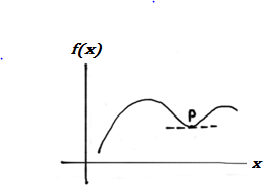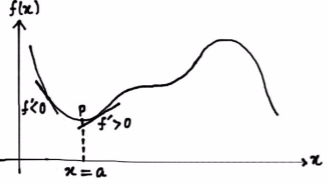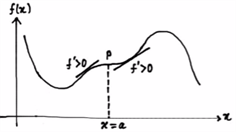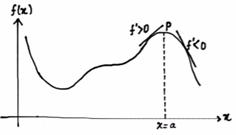Checkout JEE MAINS 2022 Question Paper Analysis : Checkout JEE MAINS 2022 Question Paper Analysis :

# Maxima & Minima- Using First Derivative Test

Maxima and minima of a function are very important concepts which are applicable both for Mathematics and Science. This poses an obvious question, how to find them?
To start with it, let us see the first method i.e. first derivative test. This method is based on the basic concept of increasing and decreasing functions.

From the definition of the function, we can determine the critical points by f’ = 0. If at any point on the curve where x = a.f'(a) , or is not differentiable at a, then is known as a critical point. If you could find out where the function is increasing and decreasing, we can tell whether the given critical point is a local maximum or minimum. But wait, there is a catch. This first derivative test definition holds true only if the function is continuous at the point x = a, where the test is being applied. If it’s not continuous there, then you can not apply this method. Let us try to understand this with an example.

## First Derivative Test For Maxima and MinimaFigure 1: Graph with a local minimum at

In fig. 1, we can see that point

$$\begin{array}{l} P \end{array}$$
, where
$$\begin{array}{l} x = a,f'(a) = 0\end{array}$$
. What do you observe here? If you move towards just left of
$$\begin{array}{l} P\end{array}$$
, the function
$$\begin{array}{l} f \end{array}$$
is decreasing in nature and towards just right of
$$\begin{array}{l} P \end{array}$$
, it is increasing in nature. To put it in terms of derivatives, towards just left of
$$\begin{array}{l} P\end{array}$$
,
$$\begin{array}{l} f'(0) < 0 \end{array}$$
and towards just right of
$$\begin{array}{l} P \end{array}$$
,
$$\begin{array}{l} f'(0)\end{array}$$
. This is an important observation. If we go with the converse of the previous observation and find that
$$\begin{array}{l}f'< 0 ~and ~f’ > 0 \end{array}$$
towards just left and right of point
$$\begin{array}{l} P \end{array}$$
, we can conclude that point
$$\begin{array}{l} P \end{array}$$
is a local minimum. Now, what do we mean by “just left” and “just right” of point
$$\begin{array}{l} P\end{array}$$
? When we say
$$\begin{array}{l} f'(x) > 0 \end{array}$$
towards just left of point
$$\begin{array}{l} P \end{array}$$
, it means that there is a positive width
$$\begin{array}{l} h \end{array}$$
and interval
$$\begin{array}{l} (a – h, a)\end{array}$$
for which
$$\begin{array}{l} f’ > 0\end{array}$$
. Similarly, for
$$\begin{array}{l} f'(x) > 0 \end{array}$$
towards just right of
$$\begin{array}{l} P \end{array}$$
means that there is a positive width
$$\begin{array}{l} k \end{array}$$
and interval
$$\begin{array}{l} (a, a+k) \end{array}$$
for which
$$\begin{array}{l} f’> 0\end{array}$$
.

Let us assume a function which has a critical point at

$$\begin{array}{l} P(x = a) \end{array}$$
and is also continuous at
$$\begin{array}{l} x = a\end{array}$$
. We can hence conclude whatever we have discussed in three points:

• If
$$\begin{array}{l} f’ \end{array}$$
is negative towards just left of
$$\begin{array}{l} a \end{array}$$
and it is positive towards just right of
$$\begin{array}{l} a \end{array}$$
, then the point
$$\begin{array}{l} P(x = a)\end{array}$$
is a local minimum for the function
$$\begin{array}{l} f\end{array}$$
.
• If
$$\begin{array}{l} f’ \end{array}$$
is positive towards just left of
$$\begin{array}{l} a \end{array}$$
and it is negative towards just right of
$$\begin{array}{l} a \end{array}$$
, then the point
$$\begin{array}{l} P(x = a)\end{array}$$
is a local maximum for the function
$$\begin{array}{l} f\end{array}$$
.
• If
$$\begin{array}{l} f’ \end{array}$$
has same sign towards just left and just right of
$$\begin{array}{l} a \end{array}$$
, then the point
$$\begin{array}{l} P(x = a)\end{array}$$
is neither a local maximum nor a local minimum for the function
$$\begin{array}{l} f\end{array}$$
.

To summarize what we have learned, let us put it in a tabular form.

Table 1: Different cases possible for first derivative method

 Pictorial representation of f’ towards left and right of point P(In all cases, f'(a) = 0) Nature of f’ Towards just left Towards just right Whether Local maximum or minimumNegative Positive Local MinimumPositive Positive NeitherPositive Negative Local Maximum

### Example

Let us take an example to understand the application of this test. We will try to find out all the critical points for the function and tell whether they are local maxima or minima.

$$\begin{array}{l}f(x) = 0.2 x^5 + 1.25x^4 + 2x^3 + 2015^{2016}\end{array}$$

Since it is a polynomial function, it is both continuous and differentiable in its entire domain.
$$\begin{array}{l}f(x) = 0.2 x^5 + 1.25x^4 + 2x^3 + 2015^{2016}\end{array}$$

Differentiating both sides w. r. t. , we get:
$$\begin{array}{l}f'(x) = x^4 + 5x^3 + 6x^2 + 0\end{array}$$

$$\begin{array}{l}f'(x) = x^2(x^2 + 5x + 6)\end{array}$$

$$\begin{array}{l}f'(x) = x^2(x+3)(x+2)\end{array}$$
—————- (1)
For getting the critical points, we equate to 0,
$$\begin{array}{l}f'(x) = 0\end{array}$$

$$\begin{array}{l}x^2(x+3)(x+2) = 0\end{array}$$

$$\begin{array}{l}x = 0, -2, -3\end{array}$$

So, our critical points are 0, -2 and -3.

From equation 1,

$$\begin{array}{l}f'(-2.5) < 0\end{array}$$
$$\begin{array}{l}f'(-1) > 0\end{array}$$

The sign of f ‘ is changing from negative to positive as we vary from left to right of . This means that is local minimum.
$$\begin{array}{l}f'(-3.5) > 0\end{array}$$

$$\begin{array}{l}f'(-2.5) < 0\end{array}$$
The sign of  f ‘ is changing from positive to negative as we vary from left to right of . This means that is local maximum.
$$\begin{array}{l}f'(-1) > 0\end{array}$$

$$\begin{array}{l}f'(1) > 0\end{array}$$

The sign of
$$\begin{array}{l} f’ \end{array}$$
is not changing as we vary from left to right of . This means that is neither a local maximum nor a local minimum.

So, there you go! That was the first derivative test. But as it turns out, you can’t apply this for every function. For example:

$$\begin{array}{l}f(x) = x^2 + 12 sin x\end{array}$$
..
For those functions, we use a different approach that is called the second derivative test. For more information, visit BYJU’S and learn new concepts every day.